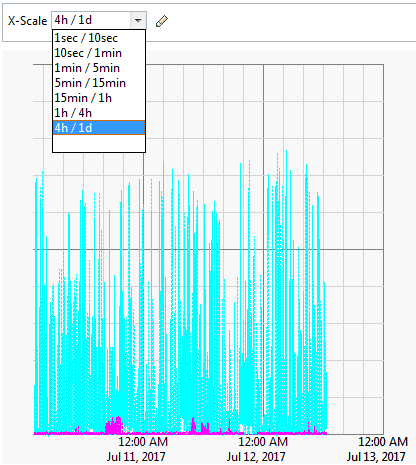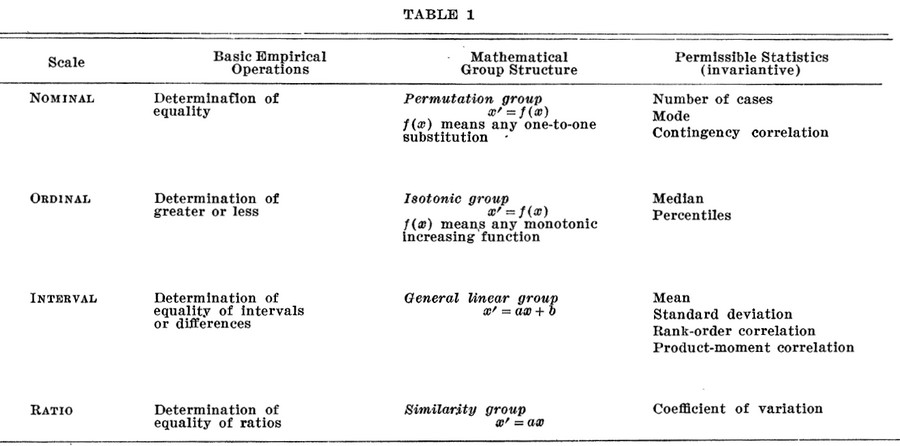# Statistics - Statistic

In general, each statistic is an estimate of a parameter, whose value is not known exactly. Every number found using a sample is just an (approximation|estimation) of a parameter (the truth).

• in descriptive statistics, a descriptive statistic is used to describe the data;
• in estimation theory, an estimator is used to estimate a parameter of the distribution (population);
• in statistical hypothesis testing, a test statistic is used to test a hypothesis.

However, a single statistic can be used for multiple purposes – for example the sample mean can be used to describe a data set, to estimate the population mean, or to test a hypothesis.

A statistic is a descriptive number::

Example:

A statistic, when used to estimate a population parameter, is called an estimator. For instance, the sample mean is a statistic that estimates the population mean, which is a parameter.

Example:

Sampling error implies that they will vary from one study to the next (from one data set to another).

A statistic describes a sample whereas a population parameter describes the population. A statistic is an observable random variable, which differentiates it both from a population parameter that is a generally unobservable quantity.

A statistic may not be representative for every individuals in the sample

The number of captured individuals is a statistic as it deals with the sample. The actual population is a parameter that we are trying to estimate.

## Parametric

A statistic is called parametric when we are trying to make inferences about population parameters, based on a sample.

## Documentation / Reference

Recommended PagesDistribution - Quantile Analysis

A quantile is a statistic that identifies the data that is less than the given value (ie that fall at or below a score in a distribution). A quantile function will always rank the data before giving any...Dos - Net Command (Network)

Net is an external command used to: update, fix, or view the network or network settings. manage Windows service manage open files synchronize time manage resources manage account and group...Metrics (Statistical Collector / Performance data)

Metrics (Statistical Collector / Performance data) This page is metrics in Monitoring. A monitoring/performance metrics is a: timestamped numerical value Monitoring Metrics are also known...Oracle Database - AWR (Advanced|Automatic) - Workload Repository - Performance Statistics Gathering Tool - DBA_HIST views

(available since Oracle 10g) AWR collects (through snapshots), processes, and maintains performance statistics. Several tools such as ADDM (Automated Database Diagnostic Monitor), AWR Reports analyses...Ordinal Data - Rank function (Ranking)

Rank is a statistic / function that calculates the rank of a value in a group of values. The position of an element in a sequence is its rank or index. This function is used in: top-N and bottom-N...Statistics

is a scientific discipline devoted to the study of data. is the art of extracting information from data. From Data to Information to Knowledge. No learning. lies lies, damned lies, and statistics....Statistics - (Data|Data Set) (Summary|Description) - Descriptive Statistics

Summary are a single value summarizing a array of data. They are: selected or calculated through reduction operations. They are an important element of descriptive analysis One of the most important...Statistics - (Estimation|Approximation)

In statistics, it's always impossible to do experimentation on the entire population. Sample of this population are then used to estimate characteristic of this population (ie the statistics). A sample...Statistics - (Estimator|Point Estimate) - Predicted (Score|Target|Outcome| )

An estimator or point estimate is a statistic that is used to infer the value of an unknown parameter in a statistical model. A point is a value in this entire possible range of values from the distribution....Statistics - (Scales of measurement|Type of variables)

According to Stevens theory (On the Theory of Scales of Measurement), the four scales of measurement are: nominal ordinal ...